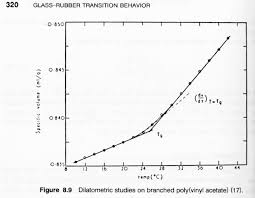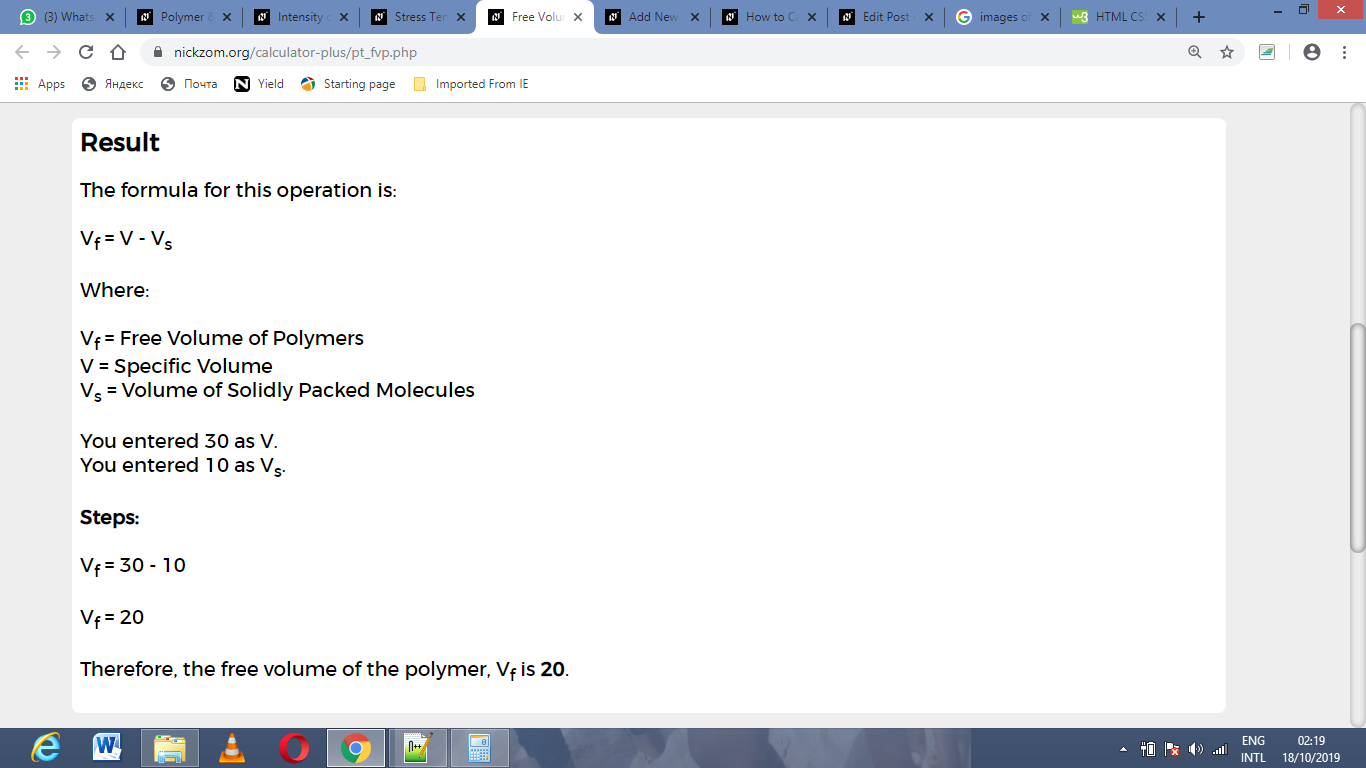# How to Calculate and Solve for Free Volume of Polymer | Polymer & TextileThe image above represents free volume of polymer.

To compute for free volume of polymer, two essential parameters are needed and these parameters are specific volume (V) and volume of solidly packed molecules (Vs).

The formula for calculating free volume of polymer:

Vf = V – Vs

Where:

Vf = Free Volume of Polymers
V = Specific Volume
Vs = Volume of Solidly Packed Molecules

Let’s solve an example;
Find the free volume of polymer when the specific volume is 30 and the volume of solidly packed molecules is 10.

This implies that;

V = Specific Volume = 30
Vs = Volume of Solidly Packed Molecules = 10

Vf = V – Vs
Vf = 30 – 10
Vf = 20

Therefore, the free volume of the polymer is 20.

Calculating the Specific Volume when the Free Volume of Polymer and the Volume of Solidly Packed Molecules.

V = Vf + Vs

Where;

V = Specific Volume
Vf = Free Volume of Polymers
Vs = Volume of Solidly Packed Molecules

Let’s solve an example;
Find the specific volume when the free volume of polymer is 24 and the volume of solidly packed molecules is 12.

This implies that;

Vf = Free Volume of Polymers = 24
Vs = Volume of Solidly Packed Molecules = 12

V = Vf + Vs
V = 24 + 12
V = 36

Therefore, the specific volume is 36.

Calculating the Volume of Solidly Packed Molecules when the Free Volume of Polymer and the Specific Volume.

Vs = V – Vf

Vs = Volume of Solidly Packed Molecules
Vf = Free Volume of Polymers
V = Specific Volume

Let’s solve an example;
Find the volume of solidly packed molecules when the free volume of polymer is 28 and the specific volume is 8.

This implies that;

Vf = Free Volume of Polymers = 28
V = Specific Volume = 8

Vs = V – Vf
Vs = 28 – 8
Vs = 20

Therefore, the volume of solidly packed molecules is 20.

Nickzom Calculator – The Calculator Encyclopedia is capable of calculating the free volume of polymer.

To get the answer and workings of the free volume of polymer using the Nickzom Calculator – The Calculator Encyclopedia. First, you need to obtain the app.

You can get this app via any of these means:

To get access to the professional version via web, you need to register and subscribe for NGN 1,500 per annum to have utter access to all functionalities.
You can also try the demo version via https://www.nickzom.org/calculator

Apple (Paid) – https://itunes.apple.com/us/app/nickzom-calculator/id1331162702?mt=8
Once, you have obtained the calculator encyclopedia app, proceed to the Calculator Map, then click on Polymer & Textile under Engineering.Now, Click on Free Volume of Polymer under Polymer & TextileThe screenshot below displays the page or activity to enter your values, to get the answer for the free volume of polymer according to the respective parameters which are the specific volume (V) and volume of solidly packed molecules (Vs).Now, enter the values appropriately and accordingly for the parameters as required by the specific volume (V) is 30 and volume of solidly packed molecules (Vs) is 10.Finally, Click on CalculateAs you can see from the screenshot above, Nickzom Calculator– The Calculator Encyclopedia solves for the free volume of polymer and presents the formula, workings and steps too.